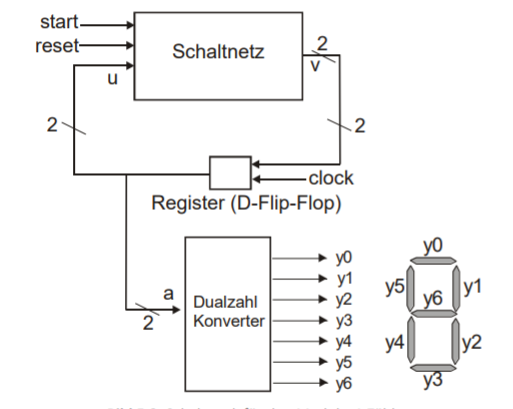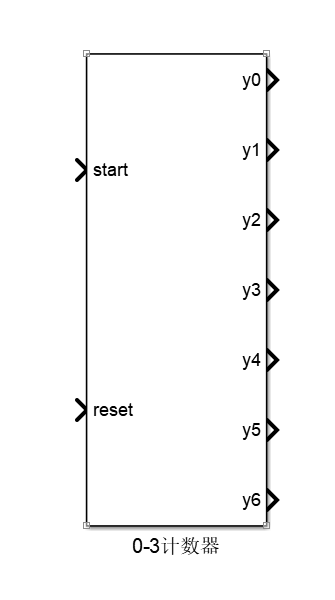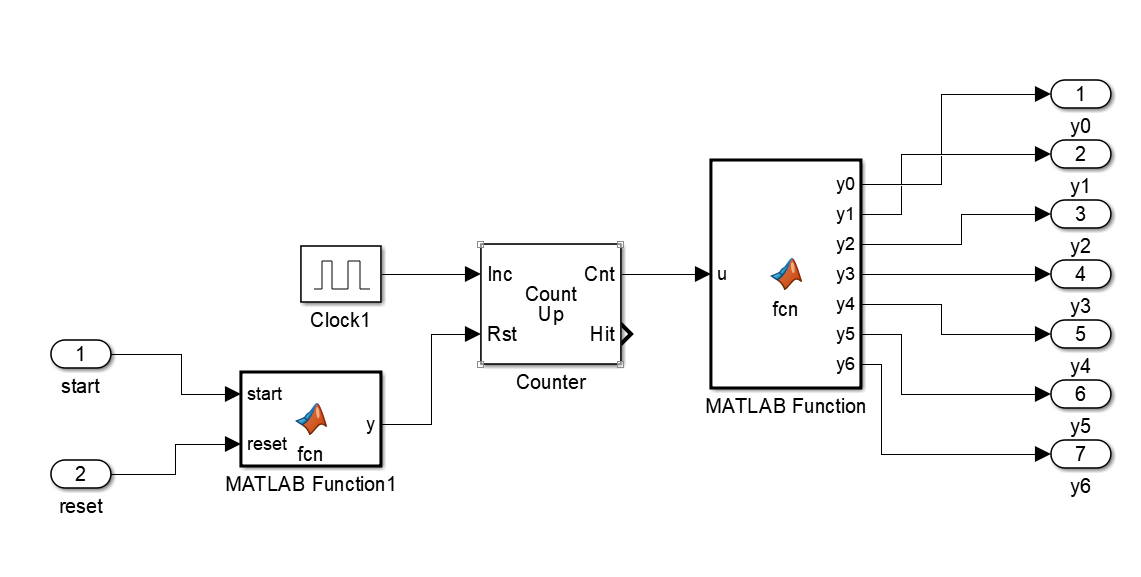###### ALbert X
2021-06-24 04:45• 点赞
• 写回答
• 关注问题
• 收藏
• 邀请回答

#### 2条回答默认 最新

•soar3033 2021-06-24 10:26
已采纳内部：matlab function1 函数定义

function y = fcn(start,reset)
y=0;
if start
y=0;
end
if reset
y=1;
end


matlab function 函数定义

function [y0,y1,y2,y3,y4,y5,y6]= fcn(u)
u=mod(floor(u),4);
if u==0
y0=1;
y1=1;
y2=1;
y3=1;
y4=1;
y5=1;
y6=0;
elseif u==1
y0=0;
y1=1;
y2=1;
y3=0;
y4=0;
y5=0;
y6=0;
elseif u==2
y0=1;
y1=1;
y2=0;
y3=1;
y4=1;
y5=0;
y6=1;

else
y0=1;
y1=1;
y2=1;
y3=1;
y4=0;
y5=0;
y6=1;
end

点赞 1 评论
•CSDN专家-sinjack 2021-06-24 08:03

循环计数器

function y = fcn(u)
%#codegen
while(1)
if(u==4)
u=0;
end
u=u+1;
y=u;
end

点赞 评论